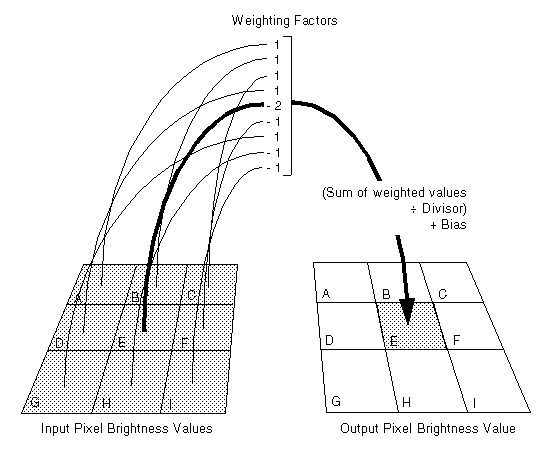﻿ SpatialFilterCommand Constructor(int,int,int[]) (SpatialFilterCommand) | Leadtools.ImageProcessing.Effects | Raster, Medical, Document Help
←Select platform
In This Topic ▼

# SpatialFilterCommand Constructor(int,int,int[])

Summary
Initializes a new SpatialFilterCommand class object with explicit parameters.
Syntax
C#
Objective-C
C++/CLI
Java
Python
````public SpatialFilterCommand( `
`   int divisor, `
`   int bias, `
`   int[] matrix `
`) ````
``- (instancetype)initWithDivisor:(NSInteger)divisor bias:(NSInteger)bias matrix:(nullable NSArray<NSNumber *> *)matrix NS_DESIGNATED_INITIALIZER; ``
````public SpatialFilterCommand( `
`   int divisor, `
`   int bias, `
`   int[] matrix `
`); ````
````public: `
`SpatialFilterCommand(  `
`   int divisor, `
`   int bias, `
`   array<int>^ matrix `
`) ````
``__init__(self,divisor,bias,matrix) # Overloaded constructor ``

#### Parameters

divisor
Number used to divide the sum of weighted values.

bias
Number added to the weighted average.

matrix
The array of weighting factors. The factors apply to pixels in the neighborhood read left to right and top to bottom, as shown below:Example

Run the SpatialFilterCommand on an image.

C#
````using Leadtools; `
`using Leadtools.Codecs; `
`using Leadtools.ImageProcessing.Effects; `
` `
` `
`public void SpatialFilterConstructorExample_S2() `
`{ `
`   // Load an image `
`   RasterCodecs codecs = new RasterCodecs(); `
`   codecs.ThrowExceptionsOnInvalidImages = true; `
` `
`   RasterImage image = codecs.Load(Path.Combine(LEAD_VARS.ImagesDir, "ImageProcessingDemo\\NaturalFruits.jpg")); `
` `
`   // Prepare the command `
`   SpatialFilterCommand command1 = new SpatialFilterCommand(SpatialFilterCommandPredefined.EmbossSouth); `
`   int[] data = command1.Matrix; `
`   SpatialFilterCommand command = new SpatialFilterCommand(command1.Divisor, command1.Bias, data); `
`   //Apply the filter. `
`   command.Run(image); `
` `
`} `
` `
`static class LEAD_VARS `
`{ `
`   public const string ImagesDir = @"C:\LEADTOOLS22\Resources\Images"; `
`} ````
Requirements